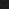JavaPerspective.com  >   Beginner Tutorials  >   5. Java in Practice  >   5.5. Working with dates

5.5. Working with dates
Last updated: 27 January 2013.

This tutorial will show you how to manipulate dates in Java.

5.5.1. The class java.util.Date

In the Java language, a given instant is represented by the number of milliseconds since January 1, 1970, 00:00:00 GMT. That date is known as the epoch. For example, the method currentTimeMillis provided by the class System returns the current time as a value of type long representing the number of milliseconds since the epoch. The class java.util.Date wraps a variable of type long representing a given instant. The following statement creates a Date object representing the current instant:

Date now = new Date();

You can compare two dates with the method compareTo(Date anotherDate) provided by the class java.util.Date. For example, the method compareDates in the class DateTesting shown below compares two dates:

import java.util.Date;

public final class DateTesting {

public void compareDates(){

Date date1 = new Date();
System.out.println
(date1);

loop
(30000);

Date date2 =
new Date();
System.out.println
(date2);

if(date1.compareTo(date2) < 0)

System.out.println(date1 + " is before " + date2);

else if(date1.compareTo(date2) == 0)

System.out.println(date1 + " is equal to " + date2);

else

System.out.println(date1 + " is after " + date2);

}

private void loop(int maxLoops){

String s = "";

// concatenating strings with the operator + in order to spend time before returning to the caller

for(int i=0; i<maxLoops; ++i)

s = s + String.valueOf(i);

}
}

5.5.2. The class Calendar

The class Calendar wraps a Date object and has additional fields as the year, month, day, hour, minute, second and millisecond. Consequently, you can get the year, month, day, hour, minute, second and millisecond of a given calendar object. You can also change the values of those fields as needed. The following statement declares a calendar object whose fields have been set to the current date and time:

Calendar now = Calendar.getInstance();

The fields of the calendar object now can be obtained by calling the method get(int field) which returns an integer value as shown below:

now.get(Calendar.YEAR)                   // returns the year
now.get(Calendar.MONTH)                // returns the month (ranges from 0 to 11)
now.get(Calendar.DATE)                   // returns the day of the month (ranges from 1 to 31)
now.get(Calendar.DAY_OF_MONTH// returns the day of the month (same as now.get(Calendar.DATE))
now.get(Calendar.HOUR_OF_DAY)    // returns the hour in the 12-hour clock (ranges from 0 to 11)
now.get(Calendar.HOUR)                  // returns the hour in the 24-hour clock (ranges from 0 to 23)
now.get(Calendar.MINUTE)              // returns the minute in the hour (ranges from 0 to 59)
now.get(Calendar.SECOND)              // returns the second in the minute (ranges from 0 to 59)
now.get(Calendar.MILLISECOND)     // returns the millisecond in the second (ranges from 0 to 999)

You can get the Date object from the calendar object now by calling the method getTime:

now.getTime() // returns a Date object representing the time value of the calendar "now"

You can also change the values of the calendar fields by calling one of the following methods: add, set or roll. For example, you can call those methods on the calendar object now like this:

now.add(Calendar.MONTH, 2)                     // adds 2 months to the MONTH field, changing the YEAR field if necessary
now.set(Calendar.MONTH, Calendar.MAY)    // sets the MONTH field to MAY (value = 4)
now.roll(Calendar.DAY_OF_MONTH, 15);    // adds 15 days to the DAY_OF_MONTH field without changing larger fields

Note that the method setTime(Date date) allows you to set a calendar's time with the given date object.

5.5.3. The class GregorianCalendar

The class GregorianCalendar is a direct subclass of the class Calendar. It represents a Gregorian calendar and provides a method named isLeapYear(int year) which returns a boolean indicating whether the given year is a leap year or not.

5.5.4. The class SimpleDateFormat

The class SimpleDateFormat is a subclass of the class DateFormat and is used to parse and format dates. The simplest way of printing a date to the standard output without any formatting pattern is shown below:

Date now = new Date();
System.out.println
(now);

The last statement shown above calls the method toString provided by the class java.util.Date. However, you may need to format the date differently. The class SimpleDateFormat allows you to specify a pattern for the date you want to format. For example, the following pattern is used to concatenate the year (4 digits), the month (2 digits) and the day (2 digits):

yyyyMMdd

The API documentation of the class SimpleDateFormat shows the list of all the pattern letters you can use. As an example, here is how to print the current date to the standard output using the pattern yyyyMMdd:

import java.text.SimpleDateFormat;
import java.util.Date;

public final class DateTesting {

public void printDate(){

Date now = new Date();
SimpleDateFormat sdf =
new SimpleDateFormat("yyyyMMdd");
String s = sdf.format
(now);
System.out.println
(s);

}
}

The class SimpleDateFormat also lets you to parse a string representing a date and get a Date object by calling the method parse(String source).

5.5.5. Code exercise

Write a method named stringToDate(String value, String pattern) that returns a Date object obtained by parsing the given string value with the given pattern.

import java.text.ParseException;
import java.text.SimpleDateFormat;
import java.util.Date;

public final class DateTesting {

public Date stringToDate(String value, String pattern) throws ParseException{

SimpleDateFormat sdf = new SimpleDateFormat(pattern);

return sdf.parse(value);

}
}

You are here :  JavaPerspective.com  >   Beginner Tutorials  >   5. Java in Practice  >   5.5. Working with dates
Next tutorial :  JavaPerspective.com  >   Beginner Tutorials  >   5. Java in Practice  >   5.6. JAR files